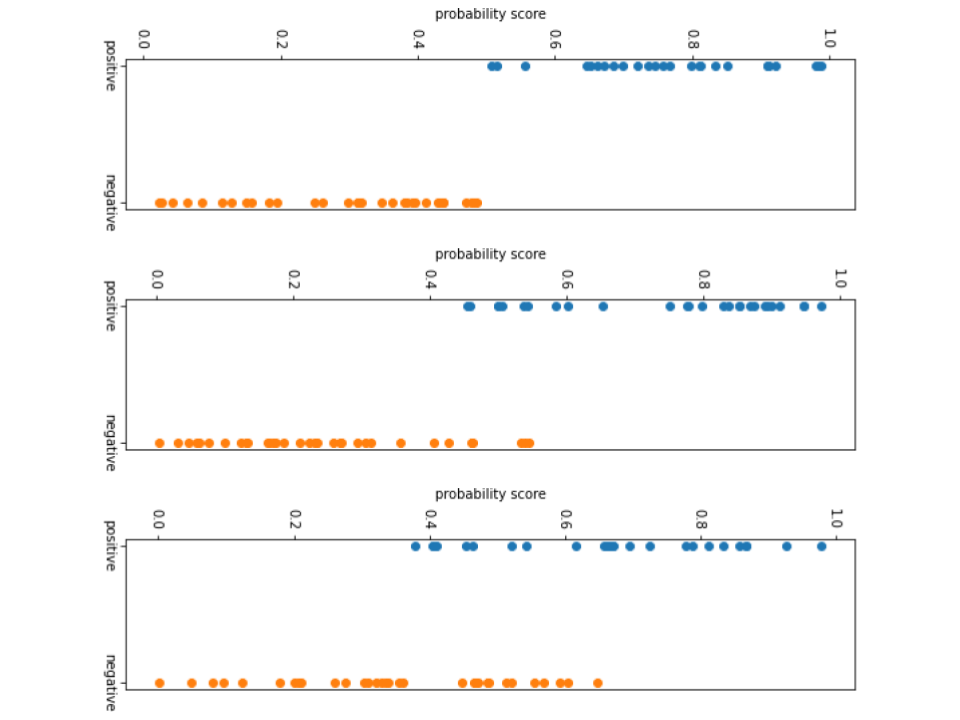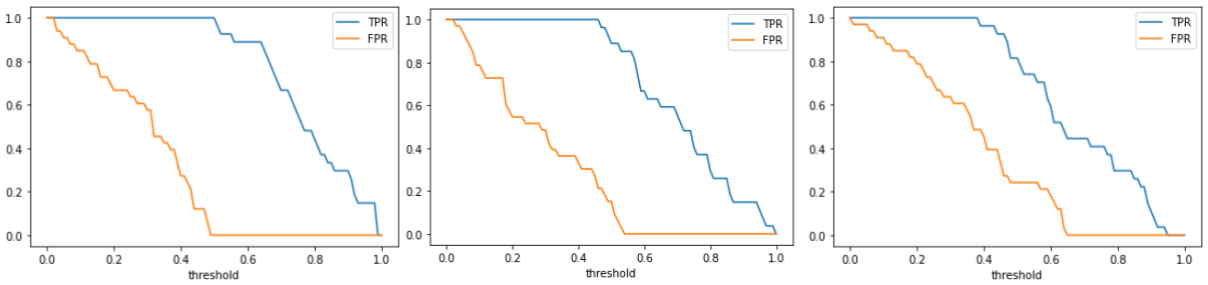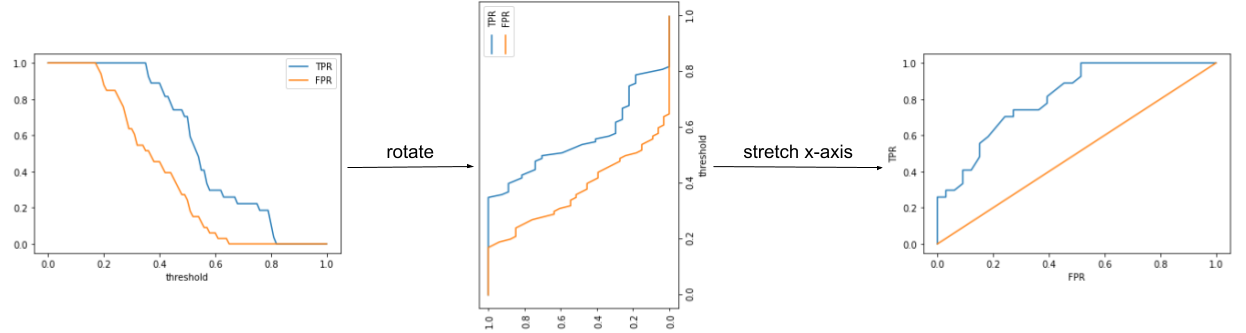# ROC curve explained

I’m not a big fan of pretentious statistical terms. Everything should have a need and use case. In this article I try to explain the ROC curve and the ROC-AUC (area under the curve) by comparing different models and developing intuition to satisfy myself.

Consider the example of testing a patient for a disease. We call it positive if the patient has the disease and negative if he doesn’t.

A true *something* is when our model predicts the truth correctly. A true positive and a true negative is when the model predicts whether a person has or doesn’t have the disease correctly.

A false *something* is when our model’s predictions are wrong. A false positive is when our model says a person has the disease when in fact he doesn’t. A false negative is when the person has the disease but our model says he’s healthy.

I know it gets messy to remember these 4 classifications. Irrespective of the actual classification you are doing, always revert back to this disease example to gain clarity on these terms.

A few more terms to know. I know it sucks but please bear with me.

True Positive Rate = TP / (TP + FN). It measures how many diseased people did our model identify among all the diseased people.

False Positive Rate = FP/(FP+TN). It measures how many of healthy people did it wrongly classify as diseased people.

ROC (Receiver Operator Characteristics) is a 2D plot used to understand how good a binary classification model is. A binary classification model in our case can be any model that provides a probability between 0 to 1 of belonging to the positive class.

Generally in binary classification problems we apply a threshold to the probability output by the model to actually classify the data points. Usually this threshold is 0.5 meaning a score ≥ 0.5 means that data point belongs to positive class if not it belong to the negative class.

Now, there is no hard and fast rule to set the threshold as 0.5. If you think about it changing the threshold directly affects the number of data points we classify as positive and negative, which in turn affects TP, FP, TN, FN, which again affects the TPR and FPR.

So let’s find out how changing the threshold affects the true positive rate and false positive rate. This is crucial to understanding ROC.

In the following experiment we compare 3 models of different classification capacity and find out what the ROC tells us about them.

Below are three plots that show the output probabilities of three different binary classification models on the same data.output probabilities of 3 different models

From a simple glance at the figure you can tell that model 1 is good at separating the positive and negative classes because there is hardly any overlap in the score. Whereas in model 2 and 3 the scores overlap, meaning whatever the threshold you choose, there will always be some data points that will be misclassified.

Given the probability scores and a threshold we can compute TP, FP, TN, FN and eventually TPR and FPR.

Now these numbers, by themselves are helpful, but not very intuitive. Let us plot these values for different values of threshold from 0 to 1.TRP and FPR vs threshold for the 3 models

Something we can immediately notice is that the area between the TPR and FPR curve reduces as the model’s classification capacity reduces. What is this area actually? Does it have a meaning rather than being just a side effect?

Area b/w TPR and FPR = Area under TPR - Area under FPR

In an ideal (best) classifier we need high TPR (possibly 1) and low FPR (possibly 0). From the graph we can treat Area under TPR as average TPR i.e E[TPR] and Area under FPR as average FPR i.e E[FPR]. Thus the Area b/w is the difference E[TPR] - E[FPR]. This difference is maximised when either TPR is high or FPR is low both of which are desirable.

Thus we can conclude that area between TPR and FPR plots is actually a good indicator of the model’s ability to separate classes.

ROC is just a modified plot of the TPR, FPR vs threshold graphs. The modification is that we remove threshold from the picture and plot only TPR vs FPR. This is equivalent to rotating the plot by 90 degrees and stretching the x-axis so that FPR curve is linear.Intuition for going from TPR, FPR vs threshold to TPR vs FPR

Vaguely the area between the TPR and FPR is proportional to the area under the ROC curve. Please look closely at the transformed plot above to ensure this yourselves. This is the reason AUC (area under the ROC curve) is used as a metric for judging the model.

Another easy way to judge a ROC plot is the more it looks like the first ROC below the better the model is. This because a model with 0 FPR and 1 TPR is the best.